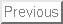Some results for nonlinear equations and systems of ode's
with p-laplacian like operators.

Raul Manásevich

Centro de Modelamiento Matemático and Departamento de Ingeniería Matemática, F.C.F.M., Universidad de Chile,
Casilla 170, Correo 3, Santiago, Chile
E-mail: manasevi@dim.uchile.cl

Let us consider the so-called one-dimensional p-Laplacian operator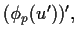where p > 1 and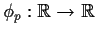is given by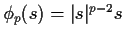for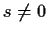and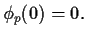Problems with separated two-point boundary value or periodic conditions containing this operator have received a lot of attention lately with respect to existence and multiplicity of solutions.

Our aim in this talk is to deal with existence of periodic solutions to some system cases involving fairly general vector-valued operator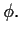Thus we will consider the boundary value problem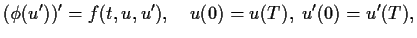(1)

where the function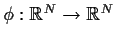satisfies some monotonicity conditions which ensure that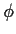is an homeomorphism onto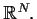As a consequence our results will apply to a large class of nonlinear operators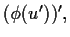which for example contain some vector versions of p-Laplacian operators like the case when for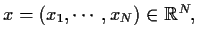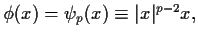for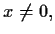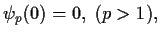and the case when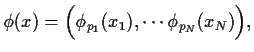with, for each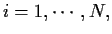pi>1, and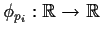is a one dimensional pi-Laplacian.